## Example Questions

### Example Question #1 : How To Find The Height Of A Triangle

A triangle has a base of 5 cm and an area of 15 cm. What is the height of the triangle?

5 cm

3 cm

1.5 cm

6 cm

None of the above

6 cm

Explanation:

The area of a triangle is (1/2)*base*height. We know that the area = 15 cm, and the base is 5 cm, so:

15 = 1/2 * 5 * height

3 = 1/2 * height

6 = height

### Example Question #2 : How To Find The Height Of A Triangle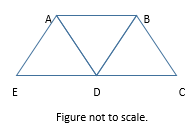In the figure above, AB = AD = AE = BD = BC = CD = DE = 1. What is the distance from A to C?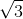Explanation: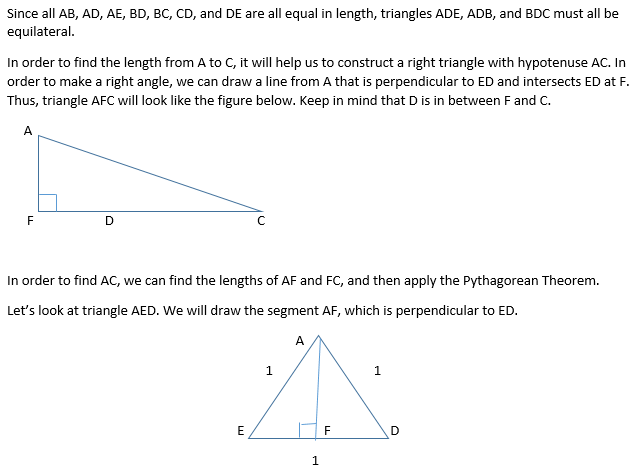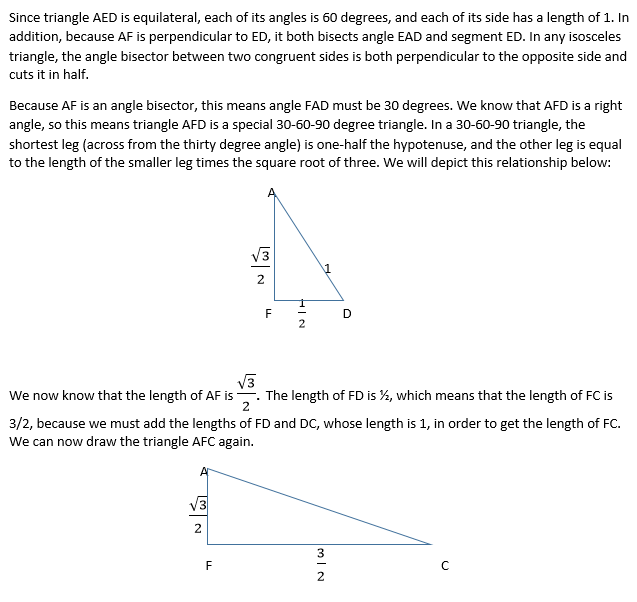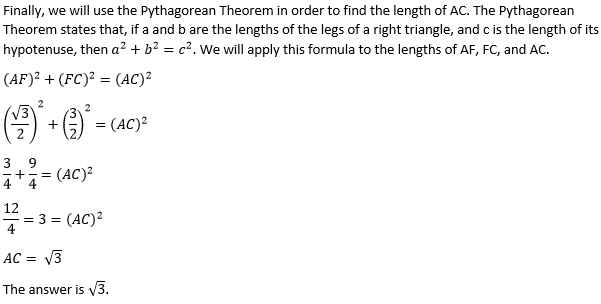### Example Question #3 : How To Find The Height Of A Triangle

A triangles has sides of 5, 9, and. Which of the folowing CANNOT be a possible value of?Explanation:

The sum of the lengths of the shortest sides of a triangle cannot be less than the third side.

3 + 5 = 8 < 9, so 3 can't be a value of x.

### Example Question #4 : How To Find The Height Of A Triangle

An equilateral triangle has a side length of 4. What is the height of the triangle?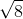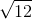Explanation:

Because the triangle is an equilateral triangle, you know that all sides have the same length, so all sides have the length of 4. Draw the triangle and label all sides as 4. Next, draw a point in the middle of one of the sides, and label each side as 2. Draw a line segment from the midpoint that you just created to the opposite angle of the triangle. That line segment is the height of the triangle. You can solve for it by using the Pythagorean theorem.

The Pythagorean theorem states thatIn our scenario a = 2, c = 4, and you are solving for b. After plugging the numbers into the formula you get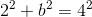and then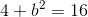and then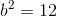and then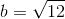Therefore, the height of the triangle is